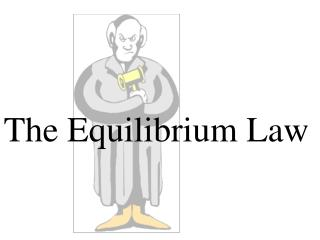# The Equilibrium Law - PowerPoint PPT PresentationDownload PresentationThe Equilibrium Law

The Equilibrium Law
Download Presentation## The Equilibrium Law

- - - - - - - - - - - - - - - - - - - - - - - - - - - E N D - - - - - - - - - - - - - - - - - - - - - - - - - - -
##### Presentation Transcript

1. The Equilibrium Law

2. Writeequilibrium lawexpressions from balanced chemical equations for heterogeneous and homogeneous systems. • Use the value of the equilibrium constant to explain how far a system at equilibrium has gone towards completion. Additional KEY Terms

3. aA + bB cC + dD Law of mass action (equilibrium law): A ratio to show the relationship between [reactants] and [products] at equilibrium. The value of this ratio is called the equilibrium constant.

4. aA + bB cC + dD If the forward and reverse reactions were elementary reactions, the rate laws would be: ratereverse = kr [C]c[D]d rateforward = kf [A]a[B]b At equilibrium rates are equal: kf [A]a[B]b = kr [C]c[D]d

5. kf Products [C]c[D]d [C]c[D]d Kc Kc = = = kr Reactants [A]a[B]b [A]a[B]b The ratio of rate constants was condensed to one constant, Kc, called the equilibrium constant. The law of mass action or equilibrium law then becomes

6. Writing the Equilibrium Law [NH3]2 Kc = [N2][H2]3 N2 (g) + 3 H2 (g) 2 NH3 (g) Homogeneous equilibria: Reactants and products are all in either gas (g) or aqueous (aq) phase.

7. Heterogeneous equilibria - reactants and products in more than one state. When writing the mass action expression, omit solids (s) or liquids (l). C(s) + H2O(g) CO(g) + H2(g) Kc [CO][H2] = [H2O] Concentration of solids and liquids don't change at constant temperatures.

8. The Equilibrium Constant

9. As with most constants, the only factor affecting Kc is temperature. PCc PDd [C]c[D]d Kc Kp = = [A]a[B]b PAa PBb The Kc can indicate whether there are more products or reactants at equilibrium:

10. Case 1: If Kc = 1[products] = [reactants] aA + bBcC + dD Concentration Rate te te

11. Case 2: If Kc > 1[product] > [reactant] Products are favoured. aA + bBcC + dD Rate Concentration te te

12. Case 3: If Kc < 1[product] < [reactant] Reactants are favoured. aA + bBcC + dD Rate Concentration te te

13. Most chemical reactions are reversible. • Chemical equilibrium can only occur in a closed system. • Equilibrium is when rates are equal AND the • [ ]s remains constant. • Kc can be used to determine the equilibrium position.

14. CAN YOU / HAVE YOU? • Writeequilibrium lawexpressions from balanced chemical equations for heterogeneous and homogeneous systems. • Use the value of the equilibrium constant to explain how far a system at equilibrium has gone towards completion. Additional KEY Terms